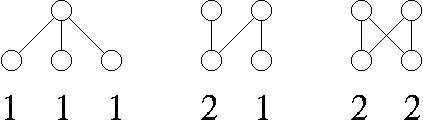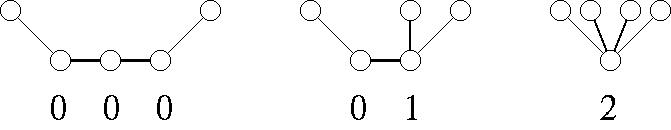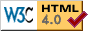## Graph Catalogs

In this page, we provide graph catalogs of the following graph classes:

### Chain graphs

The list provides all nonisomorphic connected chain graphs of n vertices for n=1,2,...,24. The numbers of graphs are summarized as follows
 The number of vertices The number of connected chain graphs (see OEIS:A051437): 1 2 3 4 5 6 7 8 9 10 11 12 13 14 15 16 17 18 19 20 21 22 23 24 1 1 1 3 4 10 16 36 64 136 256 528 1024 2080 4096 8256 16384 32896 65536 131328 262144 524800 1048576 2098176
Each line contains a sequence of degrees of one of bipartite set. That is, the number of chain graphs of 4 vertices is three and they are given by [1,1,1], [2,1], and [2,2] as follows:### Caterpillars

The list provides all nonisomorphic caterpillars of n vertices for n=1,2,...,24. The numbers of graphs are summarized as follows
 The number of vertices The number of caterpillars (see OEIS:A005418): 1 2 3 4 5 6 7 8 9 10 11 12 13 14 15 16 17 18 19 20 21 22 23 24 1 1 1 2 3 6 10 20 36 72 136 272 528 1056 2080 4160 8256 16512 32896 65792 131328 262656 524800 1049600
Each line contains a sequence of degrees of the backbone of a caterpillar. We note that we assume that the endpoints of the backbone has one leaf as a default, and these leaves are not counted. That is, the number of caterpillars of 5 vertices is three and they are given by [0,0,0], [0,1], and  as follows:### Distance hereditary graphs

The lists provide all nonisomorphic distance hereditary graphs of n vertices for n=1,2,...,14. The numbers of graphs are summarized as follows
 The number of vertices The number of distance hereditary graphs (see OEIS:A277862): 1 2 3 4 5 6 7 8 9 10 11 12 13 14 1 1 2 6 18 73 308 1484 7492 40010 220676 1253940 7282316(Note:zipped in 39MB, but 902MB if unzipped.) 43096792(Note:zipped in 212MB, but 3.2GB if unzipped.)
Each distance hereditary graph is represented in the following form:
```n m
list of edges.
```
For example, the set of distance hereditary graphs of three vertices is descirbed as
```3 2
0 1
0 2
3 3
0 1
0 2
1 2
```
The first graph has 3 vertices with 2 edges {0,1} and {0,2}, and The second graph has 3 vertices with 3 edges {0,1} and {0,2}, and {1,2}.

### Ptolemaic graphs

The lists provide all nonisomorphic Ptolemaic graphs of n vertices for n=1,2,...,15. The numbers of graphs are summarized as follows
 The number of vertices The number of Ptolemaic graphs (see OEIS:A287888): 1 2 3 4 5 6 7 8 9 10 11 12 13 14 15 1 1 2 5 14 47 170 676 2834 12471 56675 264906 1264851 6150187 30357300(Note:zipped in 292MB, but 3.8GB if unzipped.)
Each Ptolemaic graph is represented in the following form:
```n m
list of edges.
```
For example, the set of distance hereditary graphs of three vertices is descirbed as
```3 2
0 1
0 2
3 3
0 1
0 2
1 2
```
The first graph has 3 vertices with 2 edges {0,1} and {0,2}, and The second graph has 3 vertices with 3 edges {0,1} and {0,2}, and {1,2}. (As same as the set of distance hereditary graphs up to there. Please remind that the set of Ptolemaic graphs is the intersection of the sets of chordal graphs and distance hereditary graphs.)

### Interval graphs

The lists provide all nonisomorphic interval graphs of n vertices for n=1,2,...,14. The numbers of graphs are summarized as follows
 The number of vertices The number of interval graphs: The number of connected interval graphs: LIST, LIST, OEIS:A005975 OEIS:A005976 1 2 3 4 5 6 7 8 9 10 11 12 13 14 1 2 4 10 27 92 369 1807 10344 67659 491347 3894446 1 1 2 5 15 56 250 1328 8069 54962 410330 3317302
Each interval graph is represented in one line in a simple text format, e.g., 1 1 2 2 3 3 (independent set of three vertices), 1 2 1 3 2 3 (path of length 3), 1 2 3 3 2 1 (complete graph of size 3).

### Permutation graphs

The lists provide all nonisomorphic permutation graphs of n vertices for each of n=1,2,...,8. The numbers of graphs are summarized as follows
 The number of vertices The number of permutation graphs The number of connected permutation graphs 1 2 3 4 5 6 7 8 1 2 4 11 33 142 776 5699 1 1 2 6 20 99 600 4753
Each permutation graph represented in one line in a simple text format, e.g., 1 2 3 (independent set of three vertices), 2 3 1 (path of length 3), 3 2 1 (complete graph of size 3).

### Connected Proper Interval graphs

The list provides all nonisomorphic proper interval graphs of n vertices for n=1,2,...,23. The numbers of graphs are summarized as follows
 The number of vertices The number of connected proper interval graphs: 1 2 3 4 5 6 7 8 9 10 11 12 13 14 15 16 17 18 19 20 21 22 23 1 1 2 4 10 26 76 232 750 2494 8524 29624 104468 372308 1338936 4850640 17685270 64834550 238843660(608M) 883677784(2216M) 3282152588(8136MB) 12233309868(31GB) 45741634536(115GB)
Each interval graph is represented in one line in a simple text format, e.g., [[[]]] (complete graph of size 3), [[][]] (path of length 3).

### Histrory

• 2020/11/09: Update
• Chain graphs (n=1,2,...,24) (Thanks to Kazuaki Yamazaki.)
• 2020/11/04: Update
• Caterpillars (n=1,2,...,24) (Thanks to Kazuaki Yamazaki.)
• 2020/07/06: Update
• Ptolemaic graphs (n=1,2,...,15) (Thanks to Kazuaki Yamazaki.)
• 2020/06/12: Update
• Distance hereditary graphs (n=13,14) (Thanks to Kazuaki Yamazaki.)
• 2020/06/10: Update
• Distance hereditary graphs (n=1,2,...,12) (Thanks to Kazuaki Yamazaki.)
• 2019/09/04: Update & Bug fix
• Permutation graphs (n≤8)
• Connected permutation graphs (n≤8) (Thanks to Kazuaki Yamazaki.)
• 2019/04/01-04/11: Update;
• Connected proper interval graphs (n=21,22,23) (Special thanks to Prof. Ryuichi SAKAI at Osaka Electro-Communication University. He made a nice program for enumeration of these graphs. Note that his program can compute for n=24, 25, but they are too huge to put on the Web site.)
• 2019/03/19: Update;
• Connected proper interval graphs (n=19,20) (Special thanks to Prof. Ryuichi SAKAI at Osaka Electro-Communication University. He made a nice program for enumeration of these graphs.)
• 2019/02/23: Update;
• Connected proper interval graphs (n=1,2,...,18)
• 2019/01/09: Update;
• We add the note about bugs for permutation graphs. (Many thanks to Prof. Yota Otachi.)
• 2017/10/16: Update;
• Interval graphs (n=12)
• Connected interval graphs (n=12)
• 2017/08/24: Update;
• Interval graphs (n=11)
• Connected interval graphs (n=11)
• 2017/08/08: Update;
• Interval graphs (n=10)
• Connected interval graphs (n=10)
• 2017/07/20: Update;
• Permutation graphs (n=6)
• Connected permutation graphs (n=6)
• 2017/06/30: Open for the following graph classes;
• Interval graphs (n=1,...,9)
• Connected interval graphs (n=1,...,9)
• Permutation graphs (n=1,...,6)
• Connected permutation graphs (n=1,...,6)

 Last modified: Fri Dec 24 20:14:43 JST 2010 by Ryuhei Uehara (uehara@jaist.ac.jp)